# Week 12 Day 2 Opener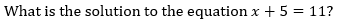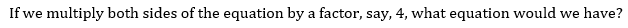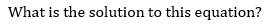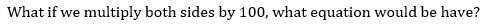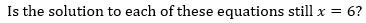Graph the equation 4x + y = 1 Multiply the entire equation by 2. Write your answer below and also graph your new equation below. Multiply the original entire equation by -5. Write your answer below and also graph your new equation below. What do you notice?